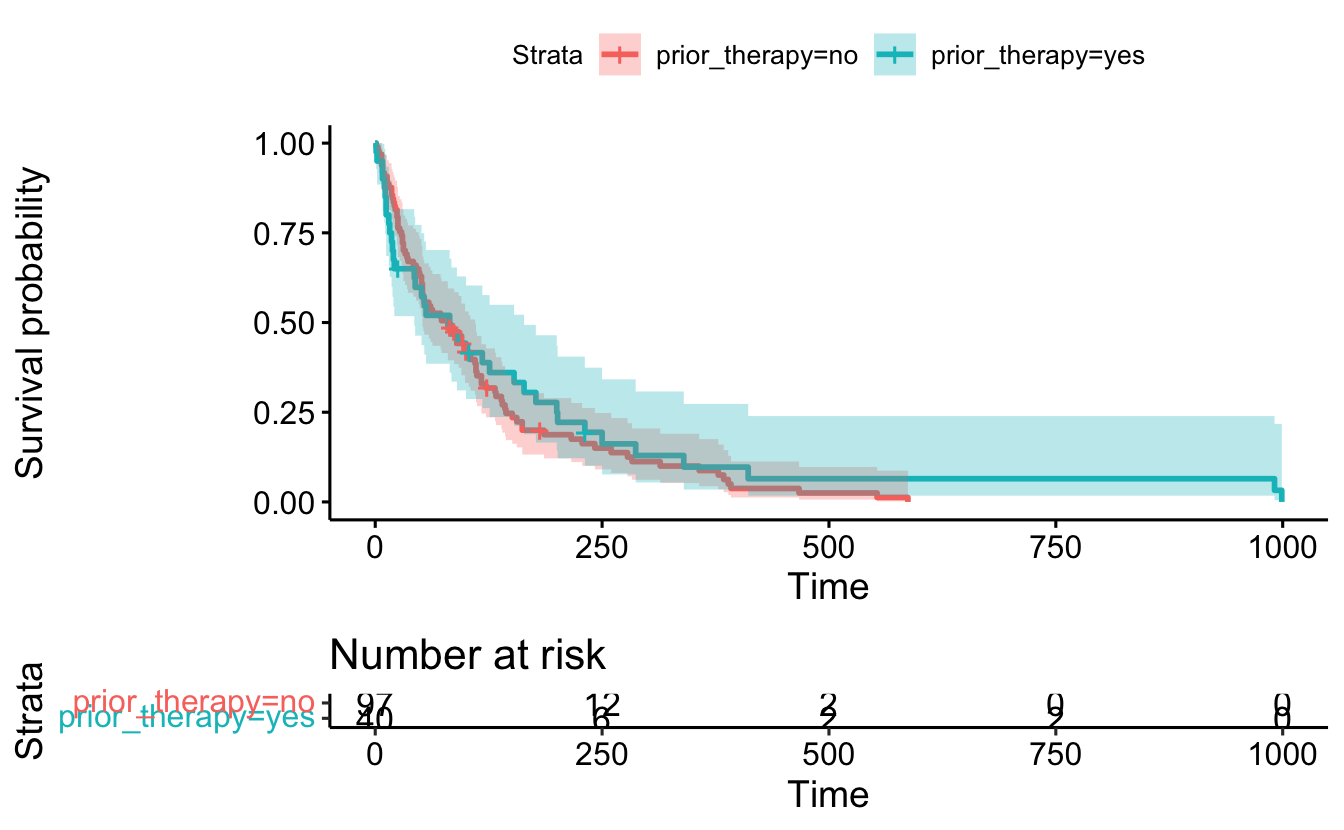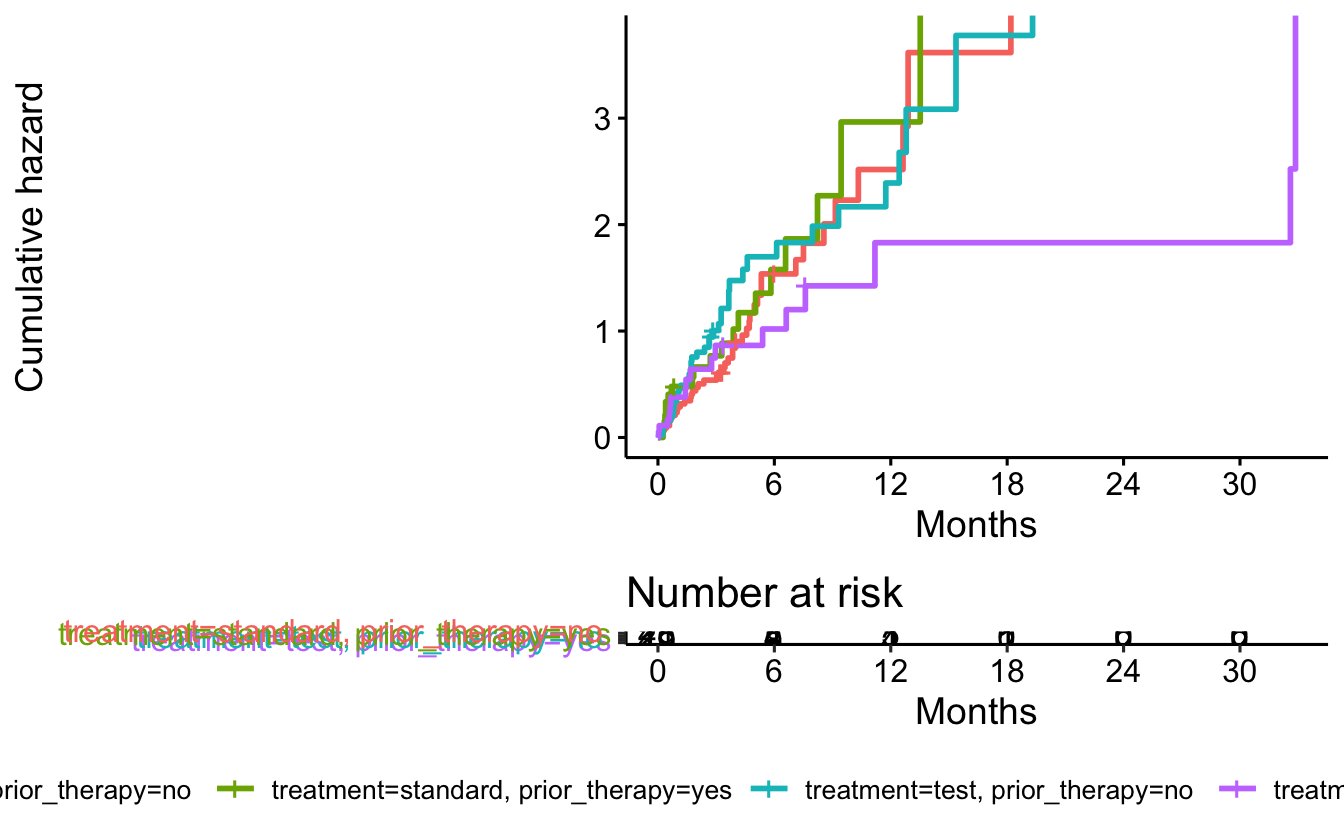# Chapter 13 Survival Analysis

https://www.emilyzabor.com/tutorials/survival_analysis_in_r_tutorial.html

over estimating survival if you don’t take into account censoring

library(tidyverse)
── Attaching packages ─────────────────────────────────────── tidyverse 1.3.1 ──
✔ ggplot2 3.3.5     ✔ purrr   0.3.4
✔ tibble  3.1.4     ✔ dplyr   1.0.7
✔ tidyr   1.1.4     ✔ stringr 1.4.0
✔ readr   2.0.2     ✔ forcats 0.5.1
── Conflicts ────────────────────────────────────────── tidyverse_conflicts() ──
✖ dplyr::lag()    masks stats::lag()
cancer <- read_csv("data/survival/veterans_lung_cancer.csv")
Rows: 137 Columns: 8
── Column specification ────────────────────────────────────────────────────────
Delimiter: ","
chr (3): celltype, prior_therapy, treatment
dbl (4): survival_in_days, age_in_years, karnofsky_score, months_from_diagnosis
lgl (1): status

ℹ Use spec() to retrieve the full column specification for this data.
ℹ Specify the column types or set show_col_types = FALSE to quiet this message.
cancer
# A tibble: 137 × 8
status survival_in_days age_in_years celltype karnofsky_score
<lgl>             <dbl>        <dbl> <chr>              <dbl>
1 TRUE                 72           69 squamous              60
2 TRUE                411           64 squamous              70
3 TRUE                228           38 squamous              60
4 TRUE                126           63 squamous              60
5 TRUE                118           65 squamous              70
6 TRUE                 10           49 squamous              20
7 TRUE                 82           69 squamous              40
8 TRUE                110           68 squamous              80
9 TRUE                314           43 squamous              50
10 FALSE               100           70 squamous              70
# … with 127 more rows, and 3 more variables: months_from_diagnosis <dbl>,
#   prior_therapy <chr>, treatment <chr>
• S(t): the survival probability of surviving beyond time, t givin they have survived until now.
library(survival)

Attaching package: 'survival'
The following object is masked _by_ '.GlobalEnv':

cancer

The survival object uses the Surv function. The + denotes a censored subject.

Surv(cancer$survival_in_days, cancer$status)
    72  411  228  126  118   10   82  110  314  100+  42    8  144   25+  11
  30  384    4   54   13  123+  97+ 153   59  117   16  151   22   56   21
  18  139   20   31   52  287   18   51  122   27   54    7   63  392   10
   8   92   35  117  132   12  162    3   95  177  162  216  553  278   12
 260  200  156  182+ 143  105  103  250  100  999  112   87+ 231+ 242  991
 111    1  587  389   33   25  357  467  201    1   30   44  283   15   25
 103+  21   13   87    2   20    7   24   99    8   99   61   25   95   80
  51   29   24   18   83+  31   51   90   52   73    8   36   48    7  140
 186   84   19   45   80   52  164   19   53   15   43  340  133  111  231
 378   49 

Create the survival curve

sf1 <- survfit(Surv(cancer$survival_in_days, cancer$status) ~ 1, data = cancer)
sf1
Call: survfit(formula = Surv(cancer$survival_in_days, cancer$status) ~
1, data = cancer)

n events median 0.95LCL 0.95UCL
[1,] 137    128     80      52     105
names(sf1)
  "n"         "time"      "n.risk"    "n.event"   "n.censor"  "surv"
 "std.err"   "cumhaz"    "std.chaz"  "type"      "logse"     "conf.int"
 "conf.type" "lower"     "upper"     "call"     
• time: time inverval start and endpoints
• surv: sruvival prbability at each time
library(survminer)
Loading required package: ggpubr

Attaching package: 'survminer'
The following object is masked from 'package:survival':

myeloma
ggsurvplot(
fit = sf1,
xlab = "Days",
ylab = "Overall survival probability")summary(sf1, times = 365.25)
Call: survfit(formula = Surv(cancer$survival_in_days, cancer$status) ~
1, data = cancer)

time n.risk n.event survival std.err lower 95% CI upper 95% CI
365     10     118     0.09  0.0265       0.0506         0.16

## 13.1 Between Groups

sf2 <- survfit(Surv(survival_in_days, status) ~ treatment, data = cancer)
summary(sf2, times = 365.25)
Call: survfit(formula = Surv(survival_in_days, status) ~ treatment,
data = cancer)

treatment=standard
time       n.risk      n.event     survival      std.err lower 95% CI
365.2500       4.0000      60.0000       0.0708       0.0336       0.0279
upper 95% CI
0.1795

treatment=test
time       n.risk      n.event     survival      std.err lower 95% CI
365.2500       6.0000      58.0000       0.1098       0.0407       0.0530
upper 95% CI
0.2272 
ggsurvplot(
fit = sf2,
data = cancer,
xlab = "Days",
ylab = "Overall survival probability",
risk.table = TRUE)## 13.2 Multiple variables

sf3 <- survfit(Surv(survival_in_days, status) ~ prior_therapy, data = cancer)
ggsurvplot(
fit = sf3,
data = cancer,
risk.table = TRUE,
conf.int = TRUE)sf4 <- survfit(Surv(survival_in_days, status) ~ treatment + prior_therapy, data = cancer)

summary(sf4, times = 365.25)
Call: survfit(formula = Surv(survival_in_days, status) ~ treatment +
prior_therapy, data = cancer)

treatment=standard, prior_therapy=no
time       n.risk      n.event     survival      std.err lower 95% CI
365.2500       3.0000      41.0000       0.0807       0.0436       0.0280
upper 95% CI
0.2326

treatment=standard, prior_therapy=yes
time       n.risk      n.event     survival      std.err lower 95% CI
3.65e+02     1.00e+00     1.90e+01     5.16e-02     5.02e-02     7.67e-03
upper 95% CI
3.47e-01

treatment=test, prior_therapy=no
time       n.risk      n.event     survival      std.err lower 95% CI
365.2500       4.0000      43.0000       0.0916       0.0432       0.0363
upper 95% CI
0.2310

treatment=test, prior_therapy=yes
time       n.risk      n.event     survival      std.err lower 95% CI
365.2500       2.0000      15.0000       0.1604       0.0944       0.0506
upper 95% CI
0.5082 
ggsurvplot(
fit = sf4,
data = cancer)## 13.3 Cox Regression

h(t): hazard, the instantaneous rate at which events occur

coxph(Surv(survival_in_days, status) ~ treatment + prior_therapy, data = cancer)
Call:
coxph(formula = Surv(survival_in_days, status) ~ treatment +
prior_therapy, data = cancer)

coef exp(coef) se(coef)      z     p
treatmenttest     0.02608   1.02643  0.18099  0.144 0.885
prior_therapyyes -0.14474   0.86525  0.20091 -0.720 0.471

Likelihood ratio test=0.54  on 2 df, p=0.7636
n= 137, number of events= 128 

### 13.3.1 Hazard Ratio

library(broom)
coxph(Surv(survival_in_days, status) ~ treatment + prior_therapy, data = cancer) %>%
tidy() %>%
mutate(hr = exp(estimate))
# A tibble: 2 × 6
term             estimate std.error statistic p.value    hr
<chr>               <dbl>     <dbl>     <dbl>   <dbl> <dbl>
1 treatmenttest      0.0261     0.181     0.144   0.885 1.03
2 prior_therapyyes  -0.145      0.201    -0.720   0.471 0.865

Taken from Emily’s page (for now) https://www.emilyzabor.com/tutorials/survival_analysis_in_r_tutorial.html

The quantity of interest from a Cox regression model is a hazard ratio (HR). The HR represents the ratio of hazards between two groups at any particular point in time.

The HR is interpreted as the instantaneous rate of occurrence of the event of interest in those who are still at risk for the event. It is not a risk, though it is commonly interpreted as such.

If you have a regression parameter β
(from column estimate in our coxph) then HR = exp(β)

.

A HR < 1 indicates reduced hazard of death whereas a HR > 1 indicates an increased hazard of death.

our treatmenttest = 1.03 implies that around 1 times as many test are dying as standard, at any given time.
out prior_therapyyes = 0.865 implies that around 0.865 times as many yesprior are dying as noprior, at any given time.

fit <- survfit(Surv(survival_in_days, status) ~ treatment + prior_therapy, data = cancer)
ggsurvplot(data = cancer,
fit = fit,
risk.table = TRUE,
fun = "cumhaz",
legend = "bottom",
legend.title = "",
xlab = "Months",
xscale = 30.4,
break.x.by = 182.4
)